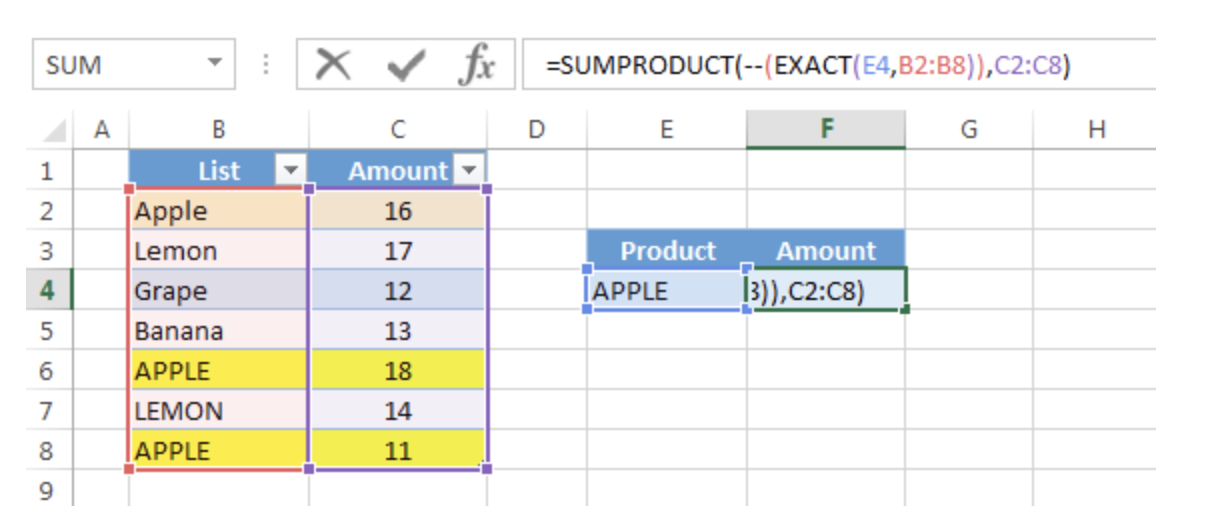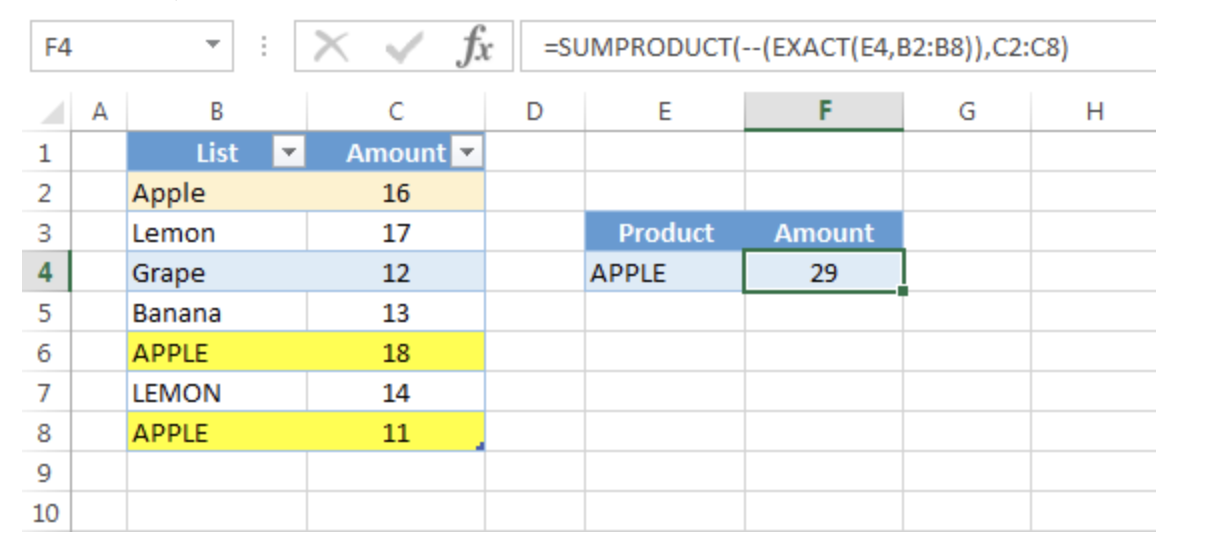Get instant live expert help with Excel or Google Sheets“My Excelchat expert helped me in less than 20 minutes, saving me what would have been 5 hours of work!”

#### Post your problem and you’ll get Expert help in seconds.

Your message must be at least 40 characters
Our professional Expert are available now. Your privacy is guaranteed.

# How to Perform Exact Match Lookup in Excel with SUMPRODUCT

The EXACT function works as a lookup tool. However, it is case-sensitive. This function compares two text strings and returns TRUE if they are exactly the same, FALSE otherwise. In this post, EXACT will be used with SUMPRODUCT function to illustrate how EXACT function works.

## Exact match lookup with SUMPRODUCT

### Generic Formula

`=SUMPRODUCT(--(EXACT( value , lookup_array)), amount_array)`

Although SUMPRODUCT works with arrays, it does not require using array shortcut (CTRL + SHIFT + ENTER). You can complete a SUMPRODUCT formula by simply pressing ENTER after typing the formula.

### `Example`

Considering the example below, the formula in D5 is:

`=SUMPRODUCT(--(EXACT(E4,B2:B8)),C2:C8)`Figure 1 – Exact match lookup with SUMPRODUCT

In this case, standard lookups like VLOOKUP of HLOOKUP will ignore case and return the first match, which is “Apple”. However, the EXACT function will match the exact cases in the lookup_array. The result will be 29:Figure 2– Exact match lookup with SUMPRODUCT

### Explanation of formula

How this formula works:

`EXACT(E4,B2:B8)`

: this portion produces an array like this

`{FALSE; FALSE; FALSE; FALSE;TRUE; FALSE;TRUE}`

–       The double unary operator (–) is used to convert the array to 1 and 0. A new array is returned like this: {0;0;0;0;1;0;1}

You can also produce 1 and 0 arrays in this way: `EXACT(E4,B2:B8)*1`

–       SUMPRODUCT then simply multiples the items in two array: {0;0;0;0;1;0;1} and {16;17;12;13;18;14;11}. The returned array is: {0;0;0;0;18;0;11}. Finally, it sums all the values and returns 28.

### Note:

• This formula will only work with numeric values. SUMPRODUCT doesn’t retrieve text.
• All arrays in SUMPRODUCT formula must have the same number of rows and columns otherwise you will get the #VALUE! error

Still need some help with Excel formatting or have other questions about Excel? Connect with a live Excel expert here for some 1 on 1 help. Your first session is always free.

### Did this post not answer your question? Get a solution from connecting with the expert.Another blog reader asked this question today on Excelchat:
Solution examplesI filtered data in column A (it is labeled ID #) of a data set. On my next worksheet, column A is also the ID #, but it is a different data set. I want to filter the ID #'s the same for the two sheets, but how do I do that being that I am working with two different sets of data?
Solved by V. H. in 22 minsI'm looking for a formula or format for if there is data in c4 on a sheet... It adds extra information underneath
Solved by X. E. in 20 minshi, my vlookup wont work in some rows but does in others. its temperemental. e.g it will work on rows 10 to 15, then not row 16 and 17, but the will for the rest.
Solved by K. Q. in 21 minsVLOOKUP help. Trying to input data from database into new table
Solved by S. Q. in 40 minsI need to count number of dates from a timestamped date in column from the completed date column to show the number of days. I have not successfully been able to do this. I am a novice Excel user. Can you help?
Solved by O. B. in 15 mins## Subscribe to Excelchat.coAnother blog reader asked this question today on Excelchat: